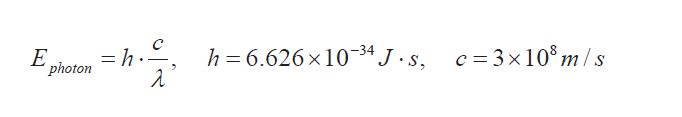Connect with us
Course Content
Atoms
Atoms are the smallest units of matter that still retain the fundamental chemical properties of an element. Much of the study of chemistry, however, involves looking at what happens when atoms combine with other atoms to form compounds. A compound is a distinct group of atoms held together by chemical bonds.
0/3
Atoms and Electron
Atoms consist of three basic particles: protons, electrons, and neutrons. The nucleus (center) of the atom contains the protons (positively charged) and the neutrons (no charge). The outermost regions of the atom are called electron shells and contain electrons (negatively charged).
0/3
Atoms

In section J. 1.2 Electron movement and electromagnetic radiation electromagnetic radiation is
described as continuous waves for which the correlation between wavelength and frequency is given
by equation (1- 1). With this opinion of electromagnetic radiation, energy portions of arbitrary size are
able to be transported by electromagnetic radiation. Howver, the German physicist Max Planck
disproved this statement by doing different experiments. He showed that energy is quantized which
means that energy on/y can be transported in portions with specific amounts of energy called
quantums. Albert Einstein further developed the theory of Planck and stated that a// electromagnetic
radiation is quantized. This means that electromagnetic radiation can be considered as a stream of very
small “particles” in motion called photons. The energy of a photon is given by equation in which is the Planck’s constant and c is the speed of light.